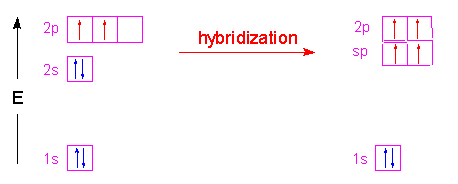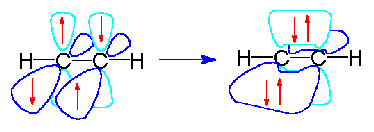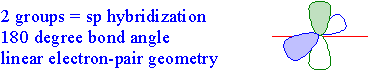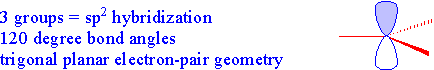Hybridization

Valence Bond Theory:

Valence bond theory is an empirically derived theory that describes how orbitals overlap in molecules to form bonds. When the bond forms, the probabiity of finding electrons changes to become higher within the region of space between the two nuclei. This simply means that electron density is highest along the axis of the bond. Single covalent bonds that form between nuclei are created from the "head-to-head" overlap of orbitals and are called sigma (s) bonds. This overlap may involve s-s, s-p, s-d or even p-d orbitals. Another type of bond, a pi (p) bond is formed when two p orbitals overlap. Pi bonds are found in double and triple bond structures.

Hybrid Orbitals:

Now let's talk about hybridization. What is a hybrid? Well, when you combine two things into one that is a hybrid. Scientists hybridize plants all the time to give them better taste, more resilience to disease etc. When we talk about hybrid orbitals we are visualizing what we believe must occur within a molecules bonding structure to result in the molecular structures we can see.

Here is what I mean: Carbon has an electron configuration of 1s2 2s2 2p2 There are four valence electrons in carbon's outermost shell that can bond: two s orbital electrons and 2 p orbital electrons. Now, remembering back to the atomic theory, we know that s orbitals are of lower energy than p orbitals, correct? So that means when they bond to other atoms, the p orbital electrons would form stronger (higher energy bonds) than the s orbital electrons. So in a molecule of CH4 you should see two long bonds between the s-s orbital overlaps, and two shorter bonds between the p-s orbital overlaps. So the structure would look like this:But we know this is not what methane (CH4) actually looks like. All the bond lengths and strengths in methane are roughly the same. So even though the bonds are made up of different energy orbitals they make all the same type of bonds, how can this be? Well, the way we explain it is hybridization.We take the two higher energy p orbital electrons and the two lower energy s orbital electrons and meld them into four equal energy sp3 ( 1s + 3 p orbitals = sp3) hybrid orbitals. When these sp3 hybrid orbitals overlap with the s orbitals of the hydrogens in methane, you get four identical bonds, which is what we see in nature.

Other hybridizations follow the same format.

Let's look at sp2 hybridization:

There are two ways to form sp2 hybrid orbitals that result in two types of bonding. 1) hybridization of an element with three valence electrons in its outer shell, like boron will yield three full sp2 hybrid orbitals and no left over electrons.or if the atom has more than three valence electrons in its outer shell three of the electron orbitals hybridize and one of the p orbitals remains unhybridized:It is the unhybridized p orbitals that then form pi bonds for double bonding:Now let's look at sp hybridization:

Again there are two ways to form sp hybrids. The first can be formed from an element with two valence electrons in its outer shell, like lithium:The second way is to form the hybrid orbitals from an element with more than two valence electrons in its outer shell, but leave some of those electrons unhybridized:Just as with the sp2 hybrids the unhybridized electrons can then form pi bonds. In the case of carbon, the two unhybridized p orbital electrons form two pi bonds which results in a triple bond structure:The table below summarizes the relationship between valence bond theory (hybridization) and electron pair geometry. Both of these designations can be assigned simply by counting the number of groups (bonds or lone pairs) attached to a central atom.

 Number of Groups Attached to a Central Atom Description and 3-Dimensional Shape Two Groups...spThree Groups...sp2Four Groups...sp3Five Groups...sp3dSix Groups...sp3d2